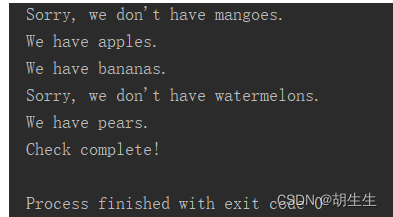### Python中 if 语句及其使用

1.比较字符串相等或不相等

1 fruit = ‘apple’
2 print(‘1.’ + str(fruit == ‘apple’)) #判断是否相等
3 print(‘2.’ + str(fruit == ‘banana’))
4 print(‘3.’ + str(fruit != ‘apple’)) #判断是否不相等
5 print(‘4.’ + str(fruit != ‘banana’))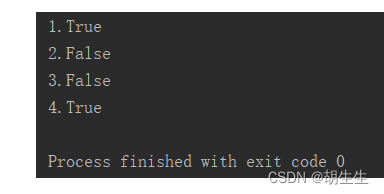2.比较数字

num1 = 20
num2 = 30
print(‘1.’ + str(num1 == num2))
print(‘2.’ + str(num1 != num2))
print(‘3.’ + str(num1 >= num2))
print(‘4.’ + str(num1 <= num2))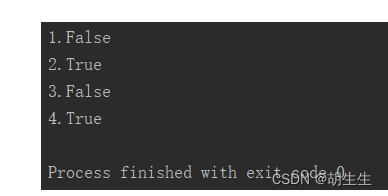3.检查多个条件

1 num1 = 20
2 num2 = 30
3 print(‘1.’ + str(num1 >= 15 and num2 >= 15))
4 print(‘2.’ + str(num1 >= 25 and num2 >= 25))
5 print(‘3.’ + str(num1 >= 25 or num2 >= 25))
6 print(‘4.’ + str(num1 >= 35 or num2 >= 35))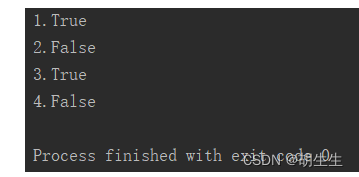4.检查特定值是否在列表中

1 fruits = [‘grape’, ‘apple’, ‘banana’, ‘orange’, ‘pear’]
2 print(‘1.’ + str(‘apple’ in fruits))
3 print(‘2.’ + str(‘mango’ in fruits))
4 print(‘3.’ + str(‘banana’ not in fruits))
5 print(‘4.’ + str(‘watermelon’ not in fruits))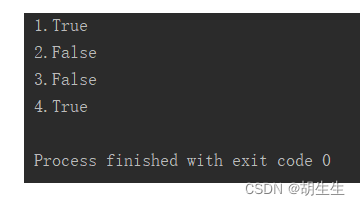1.简单的if语句

num = 25
if num >= 20:
print(‘The number is over ‘+str(num)+’.’)
if num < 20:
print(‘The number is under ’ + str(num) + ‘.’)

if语句和for循环类似，在if语句中需要执行的代码要进行缩进。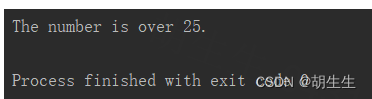2. if-else 语句

num = 25
if num >= 20:
print(‘The number is over ‘+str(num)+’.’)
else:
print(‘The number is under ’ + str(num) + ‘.’)

3. if-elif-else 语句

age = 13
if age <= 3:
print(‘The age under 3 is free.’)
elif age <= 12:
print(‘The age between 3 and 12 is half price.’)
else:
print(‘The age over 12 is full price.’)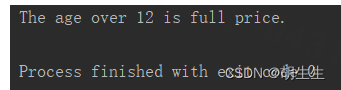1.使用 if 语句检查列表中的特殊元素

fruits = [‘grapes’, ‘apples’, ‘bananas’, ‘oranges’, ‘pears’]
for fruit in fruits:
if fruit == ‘apples’:
print(‘Sorry, we are out of apples right now.’)
else:
print(fruit.title() + ’ are available.’)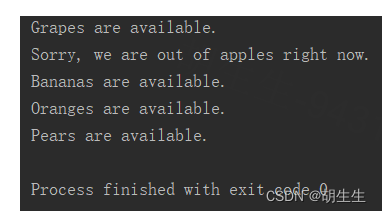2.检查列表是否为空

fruits = []
if fruits:
for fruit in fruits:
if fruit == ‘apples’:
print(‘Sorry, we are out of apples right now.’)
else:
print(fruit.title() + ’ are available.’)
else:
print(‘The list is empty.’)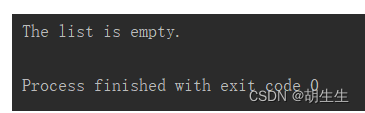3.使用多个列表

fruits_available = [‘grapes’, ‘apples’, ‘bananas’, ‘oranges’, ‘pears’]
fruits_want = [‘mangoes’, ‘apples’, ‘bananas’, ‘watermelons’, ‘pears’]
for fruit in fruits_want:
if fruit in fruits_available:
print(‘We have ’ + fruit +’.‘)
else:
print(“Sorry, we don’t have ” + fruit +’.’)
print(‘Check complete!’)